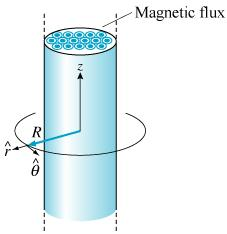1
0
watching
200
views

Faraday's law describes how electric fields and electromotive forces are generated from changing magnetic fields. This problem is a prototypical example in which an increasing magnetic flux generates a finite line integral of the electric field around a closed loop that surrounds the changing magnetic flux through a surface bounded by that loop. A cylindrical iron rod with cross-sectional area is oriented with its symmetry axis coincident with the z axis of a cylindrical coordinate system as shown. It has a uniform magnetic field inside that varies according to.1. Find, the electromotive force (EMF) around a loop that is at distance R from the z axis, where R is restricted to the region outside the iron rod as shown. Take the direction shown in the figure as positive 2. Due to the cylindrical symmetry of this problem, the induced electric fieldcan depend only on the distance R from the z axis, where R is restricted to the region outside the iron rod. Find this field